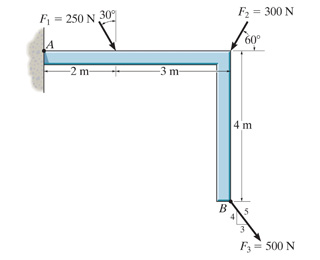# Determine the moment of each of the three forces about point BI can't figure out the moments for any of the forces given! I know that the moment is Fd, so for the first force, I set up 250sin(30)3. I get 375, which is not the answer. So I tried going the other way: 250cos(30)*4 = 866.

It's the same problem with f2 as well. I know the third one has a moment arm distance of 0, so it would be zero. the answers for f1 and f2 respectively are 150 and 600.

Any help would be appreciated!